# Interpolation with Subtable Selection Algorithm for Row-Major Array Layout

This example illustrates the algorithm for interpolating a subtable that the interpolation block selects from a higher dimension table. The interpolation algorithm with subtable selection is optimized for row-major array layout. As a reference, see the interpolation algorithm with subtable selection that is optimized for column-major array layout. The code generated by using row-major interpolation algorithm performs with the best speed and memory usage when operating on table data with row-major array layout. The code generated by using column-major algorithm performs best with column-major array layout.

In this example, you:

• Interpolate on a selected subtable with column-major and row-major algorithm.

• Preserve block semantics through table permutation.

• Generate code with row-major algorithm and array layout.

### Simulate by Using Row-Major Algorithm

1. Open example model `rtwdemo_col_interpselsubtable` and `rtwdemo_row_interpselsubtable`.

```open_system('rtwdemo_col_interpselsubtable'); open_system('rtwdemo_row_interpselsubtable'); ```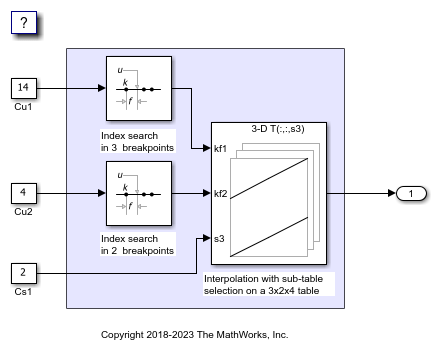2. By default, Simulink® configures a model with column-major algorithm and column-major array layout. The model `rtwdemo_col_interpselsubtable` is configured to use a column-major algorithm. Run the model and observe the output stored in workspace variable `yout`.

3. To enable row-major algorithms, open the Configuration Parameters dialog box. On the Math and Data Types pane, select the configuration parameter Use algorithms optimized for row-major array layout Alternatively, in the MATLAB® Command Window, enter:

```set_param('rtwdemo_col_interpselsubtable','UseRowMajorAlgorithm','on'); ```

4. Simulate the model and observe the error.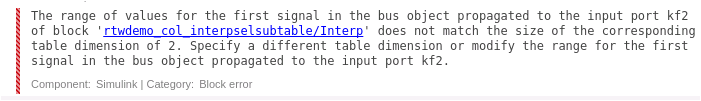The column-major and row-major algorithms differ in terms of subtable selection and interpolation order. The subtable selection is performed inside the original table. No extra memory is allocated for the subtable. The selected subtable is contiguous in memory. The interpolation order is cache-friendly for column-major algorithms with column-major array layouts and row-major algorithms with row-major array layouts. This illustration compares the row-major and column-major interpolations with subtable selection.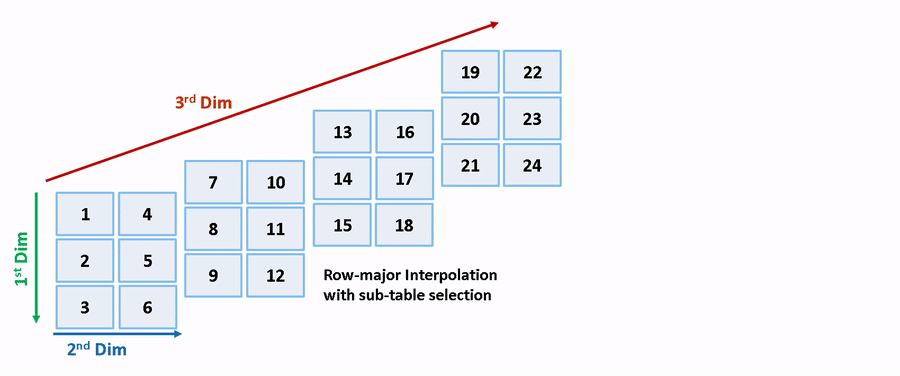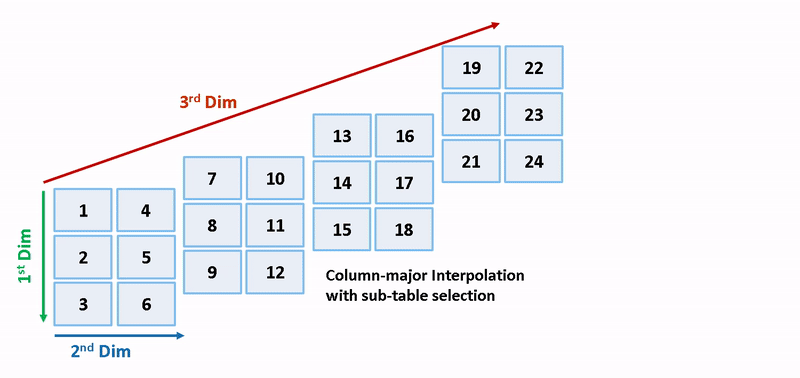Due to a change in semantics, column-major and row-major interpolations are performed on different subtables or data sets. This interpolation leads to different numeric outputs or an error.

### Preserve Semantics Through Table Permutation

With subtable selection, the model semantics change when switching from a column-major algorithm to a row-major algorithm. To preserve the semantics or ensure that the same subtable is selected for interpolation, you need to permute the table data. Otherwise, Simulink might report error if it encounters inconsistent breakpoint and table data between prelookup and interpolation blocks.

1. The block `rtwdemo_col_interpselsubtable/Interp` has 3-D table data given as T3d = reshape([1:24], 3,2,4) and one selection port with input 2 (0-based index). The selected subtable is T3d(:,:,3) (1-based index in MATLAB) for the column-major algorithm. To preserve the semantics for the row-major algorithm on the same model, that is, select the same subtable with same index and selection port inputs, permute the table as T3d_p = permute(T3d, [3,1,2]). The selected subtable is T3d_p(3,:,:) (1-based index) for the row-major algorithm.

```T3d_str = get_param('rtwdemo_col_interpselsubtable/Interp','Table'); set_param('rtwdemo_col_interpselsubtable/Interp','Table', ... ['permute(',T3d_str,',[3,1,2])']); ```

2. When you import table data from a file, you must permute the table data in the file before importing it. This permutation keeps the table tunable throughout the simulation and code generation workflow.

### Generate Code by Using Row-Major Algorithm and Array Layout

After permuting the table data, model `rtwdemo_col_interpselsubtable` is configured for row-major simulation. The model is equivalent to the preconfigured model `rtwdemo_row_interpselsubtable` that uses a row-major algorithm.

1. To set up the model for row-major code generation, open the Configuration Parameters dialog box. In addition to selecting the Use algorithms optimized for row-major array layout configuration parameter, on the Code Generation > Interface pane, set the configuration parameter Array layout to `Row-Major` option. This configuration parameter enables the model for row-major code generation. Alternatively, in the MATLAB Command Window, enter:

```set_param('rtwdemo_col_interpselsubtable', 'ArrayLayout','Row-major'); ```

2. In the dialog box, examine the permuted 3-D table data and the selected 2-D subtable.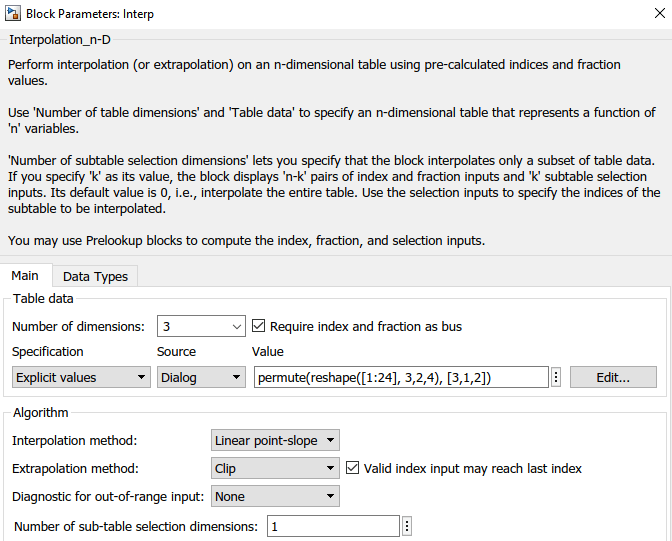3. Change your current folder in MATLAB to a writable folder. On the C Code tab, click Build to generate C code. In the generated code, observe the 2-D interpolation algorithm optimized for row-major data.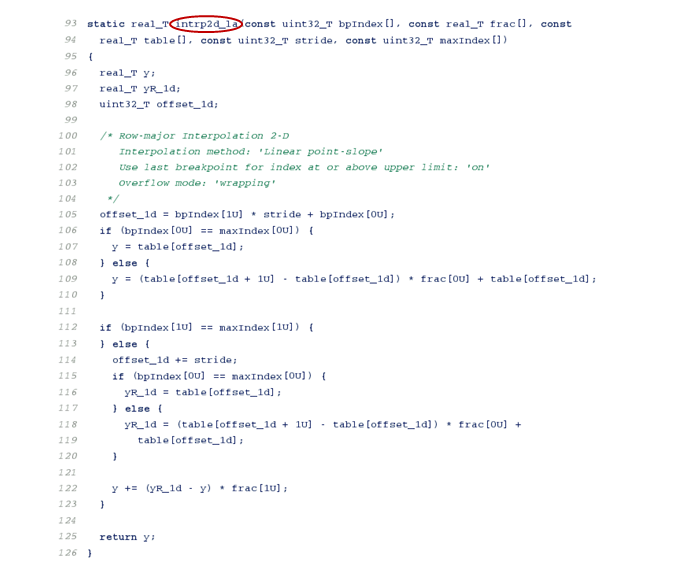```close_system('rtwdemo_col_interpselsubtable',0); ```